# Maharashtra Board 12th Commerce Maths Solutions Chapter 3 Linear Regression Miscellaneous Exercise 3

Balbharati Maharashtra State Board 12th Commerce Maths Digest Pdf Chapter 3 Linear Regression Miscellaneous Exercise 3 Questions and Answers.

## Maharashtra State Board 12th Commerce Maths Solutions Chapter 3 Linear Regression Miscellaneous Exercise 3

(I) Choose the correct alternative.

Question 1.
Regression analysis is the theory of
(a) Estimation
(b) Prediction
(c) Both a and b
(d) Calculation
(c) Both a and b

Question 2.
We can estimate the value of one variable with the help of other known variables only if they are
(a) Correlated
(b) Positively correlated
(c) Negatively correlated
(d) Uncorrelated
(a) Correlated

Question 3.
There are ________ types of regression equation
(a) 4
(b) 2
(c) 3
(d) 1
(b) 2Question 4.
In the regression equation of Y on X
(a) X is independent and Y is dependent
(b) Y is independent and X is dependent
(c) Both X and Y are independent
(d) Both X and Y are dependent.
(a) X is independent and Y is dependent

Question 5.
In the regression equation of X on Y
(a) X is independent and Y is dependent
(b) Y is independent and X is dependent
(c) Both X and Y are independent
(d) Both X and Y are dependent
(b) Y is independent and X is dependent

Question 6.
bxy is ________
(a) Regression coefficient of Y on X
(b) Regression coefficient of X on Y
(c) Correlation coefficient between X and Y
(d) Covariance between X and Y
(b) Regression coefficient of X on Y

Question 7.
byx is ________
(a) Regression coefficient of Y on X
(b) Regression coefficient of X on Y
(c) Correlation coefficient between X and Y
(d) Covariance between X and Y
(a) Regression coefficient of Y on X

Question 8.
‘r’ is ________
(a) Regression coefficient of Y on X
(b) Regression coefficient of X on Y
(c) Correlation coefficient between X and Y
(d) Covariance between X and Y
(d) Correlation coefficient between X and YQuestion 9.
bxy . byx = _________
(a) v
(b) yx
(c) r2
(d) (yy)2
(c) r2

Question 10.
If byx > 1 then bxy is ______
(a) > 1
(b) < 1
(c) > 0
(d) < 0
(b) < 1

Question 11.
|bxy + byx| > ______
(a) |r|
(b) 2|r|
(c) r
(d) 2r
(b) 2|r|

Question 12.
bxy and byx are ________
(a) Independent of change of origin and scale
(b) Independent of change of origin but not of the scale
(c) Independent of change of scale but not of origin
(d) Affected by change of origin and scale
(b) Independent of change of origin but not of the scale

Question 13.
If u = $$\frac{x-a}{c}$$ and v = $$\frac{y-b}{d}$$ then byx = ________
(a) $$\frac{d}{c} b_{v u}$$
(b) $$\frac{c}{d} b_{v u}$$
(c) $$\frac{a}{b} b_{v u}$$
(d) $$\frac{b}{a} b_{v u}$$
(a) $$\frac{d}{c} b_{v u}$$

Question 14.
If u = $$\frac{x-a}{c}$$ and v = $$\frac{y-b}{d}$$ then bxy = ________
(a) $$\frac{d}{c} b_{u v}$$
(b) $$\frac{c}{d} b_{u v}$$
(c) $$\frac{a}{b} b_{u v}$$
(d) $$\frac{b}{a} b_{u v}$$
(b) $$\frac{c}{d} b_{u v}$$Question 15.
Corr(x, x) = ________
(a) 0
(b) 1
(c) -1
(d) can’t be found
(b) 1

Question 16.
Corr (x, y) = ________
(a) corr(x, x)
(b) corr(y, y)
(c) corr(y, x)
(d) cov(y, x)
(c) corr(y, x)

Question 17.
Corr$$\left(\frac{x-a}{c}, \frac{y-b}{d}\right)$$ = -corr(x, y) if,
(a) c and d are opposite in sign
(b) c and d are same in sign
(c) a and b are opposite in sign
(d) a and b are same in sign
(a) c and d are opposite in sign

Question 18.
Regression equation of X and Y is
(a) y – $$\bar{y}$$ = byx (x – $$\bar{x}$$)
(b) x – $$\bar{x}$$ = bxy (y – $$\bar{y}$$)
(c) y – $$\bar{y}$$ = bxy (x – $$\bar{x}$$)
(d) x – $$\bar{x}$$ = byx (y – $$\bar{y}$$)
(b) x – $$\bar{x}$$ = bxy (y – $$\bar{y}$$)

Question 19.
Regression equation of Y and X is
(a) y – $$\bar{y}$$ = byx (x – $$\bar{x}$$)
(b) x – $$\bar{x}$$ = bxy (y – $$\bar{y}$$)
(c) y – $$\bar{y}$$ = bxy (x – $$\bar{x}$$)
(d) x – $$\bar{x}$$ = byx (y – $$\bar{y}$$)
Solution:
(a) y – $$\bar{y}$$ = byx (x – $$\bar{x}$$)

Question 20.
byx = ________
(a) $$r \frac{\sigma_{x}}{\sigma_{y}}$$
(b) $$r \frac{\sigma_{y}}{\sigma_{x}}$$
(c) $$\frac{1 \sigma_{y}}{r \sigma_{x}}$$
(d) $$\frac{1 \sigma_{y}}{r \sigma_{y}}$$
(b) $$r \frac{\sigma_{y}}{\sigma_{x}}$$

Question 21.
bxy = ________
(a) $$r \frac{\sigma_{x}}{\sigma_{y}}$$
(b) $$r \frac{\sigma_{y}}{\sigma_{x}}$$
(c) $$\frac{1 \sigma_{y}}{r \sigma_{x}}$$
(d) $$\frac{1 \sigma_{y}}{r \sigma_{y}}$$
(a) $$r \frac{\sigma_{x}}{\sigma_{y}}$$

Question 22.
Cov (x, y) = ________
(a) Σ(x – $$\bar{x}$$)(y – $$\bar{y}$$)
(b) $$\frac{\sum(x-\bar{x})(y-\bar{y})}{n}$$
(c) $$\frac{\sum x y}{n}-\bar{x} \bar{y}$$
(d) b and c both
(d) b and c bothQuestion 23.
If bxy < 0 and byx < 0 then ‘r’ is ________
(a) > 0
(b) < 0
(c) > 1
(b) < 0

Question 24.
If equation of regression lines are 3x + 2y – 26 = 0 and 6x + y – 31 = 0 then means of x and y are ________
(a) (7, 4)
(b) (4, 7)
(c) (2, 9)
(d) (-4, 7)
(b) (4, 7)

(II) Fill in the blanks:

Question 1.
If bxy < 0 and byx < 0 then ‘r’ is ________
negative

Question 2.
Regression equation of Y on X is ________
(y – $$\bar{y}$$) = byx (x – $$\bar{x}$$)

Question 3.
Regression equation of X on Y is ________
(x – $$\bar{x}$$) = bxy (y – $$\bar{y}$$)

Question 4.
There are ______ types of regression equations.
2

Question 5.
Corr (x1 – x) = ______
-1

Question 6.
If u = $$\frac{x-a}{c}$$ and v = $$\frac{y-b}{d}$$ then bxy = ______
$$\frac{c}{d} b_{u v}$$Question 7.
If u = $$\frac{x-a}{c}$$ and v = $$\frac{y-b}{d}$$ then byx = ______
$$\frac{d}{c} b_{v u}$$

Question 8.
|bxy + byx| ≥ ______
2|r|

Question 9.
If byx > 1 then bxy is ______
< 1

Question 10.
bxy . byx = ______
r2

(III) State whether each of the following is True or False.

Question 1.
Corr (x, x) = 1.
True

Question 2.
Regression equation of X on Y is y – $$\bar{y}$$ = bxy (x – $$\bar{x}$$).
False

Question 3.
Regression equation of Y on X is y – $$\bar{y}$$ = byx (x – $$\bar{x}$$).
True

Question 4.
Corr (x, y) = Corr (y, x).
True

Question 5.
bxy and byx are independent of change of origin and scale.
False

Question 6.
‘r’ is the regression coefficient of Y on X.
FalseQuestion 7.
byx is the correlation coefficient between X and Y.
False

Question 8.
If u = x – a and v = y – b then bxy = buv.
True

Question 9.
If u = x – a and v = y – b then rxy = ruv.
True

Question 10.
In the regression equation of Y on X, byx represents the slope of the line.
True

(IV) Solve the following problems.

Question 1.
The data obtained on X, the length of time in weeks that a promotional project has been in progress at a small business, and Y the percentage increase in weekly sales over the period just prior to the beginning of the campaign.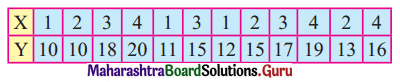Find the equation of regression line to predict percentage increase in sales if the company has been in progress for 1.5 weeks.
Solution:
Let u = x – 3, v = y – 15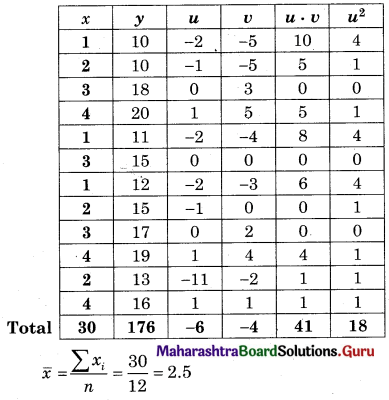∴ Regression equation of Y on X is
(y – $$\bar{y}$$) = byx (x – $$\bar{x}$$)
(y – 14.67) = 2.6(x – 2.5)
y – 14.67 = 2.6x – 6.5
y = 2.6x + 8.17
When x = 1.5
y = (2.6)(1.5) + 8.17
= 3.9 + 8.17
= 12.07

Question 2.
The regression equation of y on x is given by 3x + 2y – 26 = 0. Find byx.
Solution:
Given, regression equation of Y on X is
3x + 2y – 26 = 0
∴ 2y = -3x + 26
∴ y = $$\frac{-3}{2}$$x + 13
∴ byx = $$\frac{-3}{2}$$Question 3.
If for a bivariate data $$\bar{x}$$ = 10, $$\bar{y}$$ = 12, v(x) = 9, σy = 4 and r = 0.6. Estimate y when x = 5.
Solution:
Given, V(x) = 9
∴ σx = 3
byx = $$\frac{r \cdot \sigma_{y}}{\sigma_{x}}$$
= 0.6 × $$\frac{4}{3}$$
= 0.8
∴ Regression equation of Y on X is
(y – $$\bar{y}$$) = byx (x – $$\bar{x}$$)
(y – 12) = 0.8(5 – 10)
y – 12 = 0.8(-5)
y – 12 = -4
y = 8

Question 4.
The equation of the line of regression of y on x is v = $$\frac{2}{9} x$$ and x on y is x = $$\frac{y}{2}+\frac{7}{6}$$. Find (i) r (ii) $$\sigma_{y}^{2} \text { if } \sigma_{x}^{2}=4$$.
Solution:Question 5.
Identify the regression equations of x on y and y on x from the following equations.
2x + 3y = 6 and 5x + 7y – 12 = 0
Solution: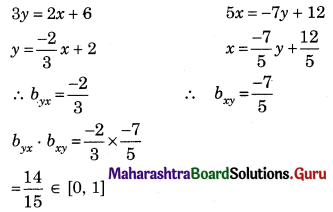∴ Our assumption is correct
∴ Regression equation of Y on X is 2x + 3y = 6
∴ Regression equation of X on Y is 5x + 7y – 12 = 0

Question 6.
(i) If for a bivariate data byx = -1.2 and bxy = -0.3 then find r.
(ii) From the two regression equations y = 4x – 5 and 3x = 2y + 5, find $$\bar{x}$$ and $$\bar{y}$$.
Solution:
r2 = byx . bxy
r2 = (-1.2) × (-0.3)
r2 = 0.36
r = ±0.6
Since, byx . bxy are negative, r = -0.6
Also,($$\bar{x}$$, $$\bar{y}$$) is the point of intersection of the regression lines
y = 4x – 5, 3x = 2y + 5
8x – 2y = 10
3x – 2y = 5
on subtracting,
5x = 5
x = 1
Substituting x = 1 in y = 4x – 5
y = 4(1) – 5
y = -1
∴ $$\bar{x}$$ = 1, $$\bar{y}$$ = -1Question 7.
The equation of the two lines of regression are 3x + 2y – 26 = 0 and 6x + y – 31 = 0. Find
(i) Means of X and Y
(ii) Correlation coefficient between X on Y
(iii) Estimate of Y for X = 2
(iv) var (X) if var (Y) = 36
Solution:
(i) Since ($$\bar{x}$$, $$\bar{y}$$) is the point of intersection of regression lines
3x + 2y = 26
6x + y = 31
3x + 2y = 26 …….(i)
12x + 2y = 62 ……..(ii)
on subtracting,
-9x = -36
x = 4
Substituting x = 4 in equation (i)
3(4) + 2y = 26
2y = 14
y = 7
∴ $$\bar{x}$$ = 4, $$\bar{y}$$ = 7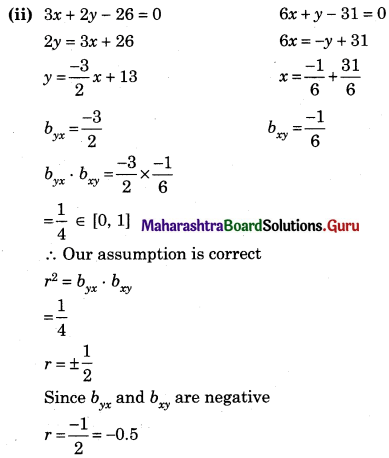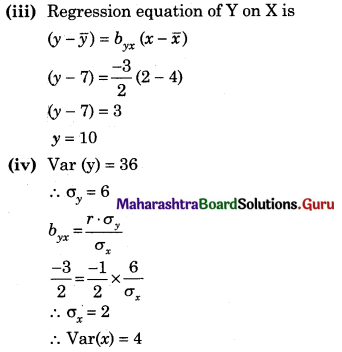Question 8.
Find the line of regression of X on Y for the following data:
n = 8, Σ(xi – x)2 = 36, Σ(yi – y)2 = 44, Σ(xi – x)(yi – y) = 24
Solution:Question 9.
Find the equation of line regression of Y on X for the following data:
n = 8, Σ(xi – $$\bar{x}$$)(yi – $$\bar{y}$$) = 120, $$\bar{x}$$ = 20, $$\bar{y}$$ = 36, σx = 2, σy = 3.
Solution: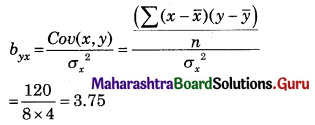Regression equation of Y on X is
(y – $$\bar{y}$$) = byx (x – $$\bar{x}$$)
(y – 36) = 3.75(x – 20)
(y – 36) = 3.75x – 75
y = 3.75x – 39Question 10.
The following result was obtained from records of age (X) and systolic blood pressure (Y) of a group of 10 men.and Σ(xi – $$\bar{x}$$)(yi – $$\bar{x}$$) = 1120. Find the Prediction of blood pressure of a man of age 40 years.
Solution: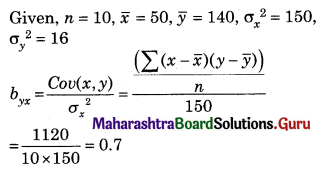Regression equation of Y on X is
(y – $$\bar{y}$$) = byx (x – $$\bar{x}$$)
(y – 140) = 0.7(40 – 50)
y – 140 = 0.7(-10)
y – 140 = -7
∴ y = 133

Question 11.
The equations of two regression lines are 10x – 4y = 80 and 10y – 9x = -40 Find:
(i) $$\bar{x}$$ and $$\bar{y}$$
(ii) byx and bxy
(iii) If var(Y) = 36, obtain var(X)
(iv) r
Solution:
(i) Since ($$\bar{x}$$, $$\bar{y}$$) is the point of intersection of regression
10x – 4y = 80 ……(i)
-9x + 10y = -40 ……..(ii)
50x – 20y = 400
-18x + 20y = -80
32x = 320
x = 10
x = 10 in equation (i)
10(10) – 4y = 80
4y = 20
y = 5
∴ $$\bar{x}$$ = 10, $$\bar{y}$$ = 5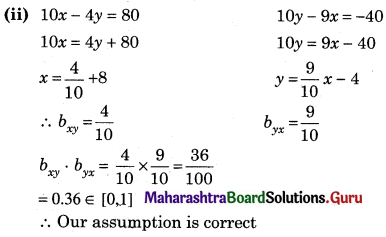(iv) r2 = byx . bxy = 0.36
r = ±0.6
Since byx and bxy are positive
∴ r = 0.6Question 12.
If byx = -0.6 and bxy = -0.216 then find correlation coefficient between X and Y comment on it.
Solution:
r2 = byx . bxy
r2 = -0.6 × -0.216
r2 = 0.1296
r = ±√0.1296
r = ± 0.36
Since byx and bxy are negative
r = -0.36